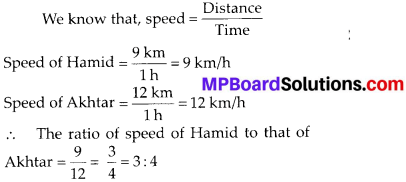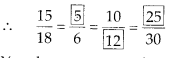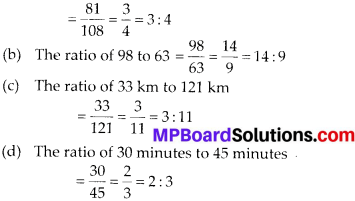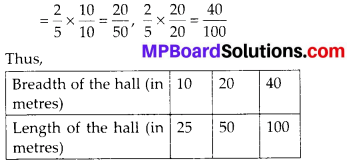# MP Board Class 6th Maths Solutions Chapter 12 Ratio and Proportion Ex 12.1

## MP Board Class 6th Maths Solutions Chapter 12 Ratio and Proportion Ex 12.1

Try this useful Ratio Simplifier and find out the simplest form of given ratio numbers.

Question 1.
There are 20 girls and 15 boys in a class.
(a) What is the ratio of number of girls to the number of boys?
(b) What is the ratio of number of girls to the total number of students in the class?
Solution:
(a) The ratio of number of girls to that of boys = $$\frac{20}{15}=\frac{4}{3}$$ = 4 : 3
(b) The ratio of number of girls to total number of students = $$\frac{20}{20+15}=\frac{20}{35}=\frac{4}{7}$$ = 4 : 7

Question 2.
Out of 30 students in a class, 6 like football, 12 like cricket and remaining like tennis. Find the ratio of
(a) Number of students liking football to number of students liking tennis.
(b) Number of students liking cricket to total number of students.
Solution:
Total number of students = 30
Number of students like football = 6
Number of students like cricket = 12
Thus, number of students like tennis = 30 – 6 – 12 = 12
(a) The ratio of number of students liking football to that of tennis = $$\frac{6}{12}=\frac{1}{2}$$ = 1 : 2
(b) The ratio of number of students liking cricket to that of total students = $$\frac{12}{30}=\frac{2}{5}$$ = 2 : 5

Question 3.
See the figure and find the ratio of
(a) Number of triangles to the number of circles inside the rectangle.
(b) Number of squares to all the figures inside the rectangle.
(c) Number of circles to all the figures inside the rectangle.Solution:
(a) The ratio of number of triangles to that of circles = $$\frac{3}{2}$$ = 3 : 2
(b) The ratio of number of squares to that of all figures = $$\frac{2}{7}$$ = 2 : 7
(c) The ratio of number of circles to that of all figures = $$\frac{2}{7}$$ = 2 : 7Question 4.
Distances travelled by Hamid and Akhtar in an hour are 9 km and 12 km. Find the ratio of speed of Hamid to the speed of Akhtar.
Solution:Question 5.
Fill in the following blanks :Solution:
In order to get the first missing number, we consider the fact that 18 = 3 × 6, i.e., we get 6 when we divide 18 by 3. This indicates that to get the missing number of the second ratio, 15 must also be divided by 3.
When we divide, we get 15 – 5 – 3 = 5. Hence, the second ratio is $$\frac{5}{6}$$ .
Similarly, to get the third ratio, we multiply both terms of the second ratio by 2. Hence, the third ratio is $$\frac{10}{12}$$.
And to get the fourth ratio, we multiply both terms of the second ratio by 5. Hence, the fourth ratio is $$\frac{25}{30}$$.Yes, these are equivalent ratios.Question 6.
Find the ratio of the following :
(a) 81 to 108
(b) 98 to 63
(c) 33 km to 121 km
(d) 30 minutes to 45 minutes
Solution:
(a) The ratio of 81 to 108Question 7.
Find the ratio of the following:
(a) 30 minutes to 1.5 hours
(b) 40 cm to 1.5 m
(c) 55 paise to Re. 1
(d) 500 ml to 2 litres
Solution:
(a) 1.5 hours = 1.5 × 60 minutes
= 90 minutes [ ∵ 1 hour = 60 minutes]
Now, the ratio of 30 minutes to 1.5 hours = 30 minutes : 1.5 hours
= 30 minutes: 90 minutes = $$\frac{30}{90}=\frac{1}{3}$$ = 1 : 3

(b) 1.5 m = 1.5 × 100 cm = 150 cm [∵ 1 m = 100 cm]
Now, the ratio of 40 cm to 1.5 m = 40 cm : 1.5 m
= 40 cm : 150 cm = $$\frac{40}{150}=\frac{4}{15}$$ = 4 : 15

(c) Re. 1 = 100 paise
Now, the ratio of 55 paise to Re. 1
= 55 paise : Re. 1 = 55 paise : 100 paise
= $$\frac{55}{100}=\frac{11}{20}$$ = 11 : 20

(d) 2 litres = 2 × 1000 ml = 2000 ml [∵ 1 litre = 1000 ml]
Now, the ratio of 500 ml to 2 litres = 500 ml : 2 litres
= 500 ml : 2000 ml = $$\frac{500}{2000}=\frac{1}{4}$$ = 1 : 4Question 8.
In a year, Seema earns Rs. 1,50,000 and saves Rs. 50,000. Find the ratio of
(a) Money that Seema earns to the money she saves.
(b) Money that she saves to the money she spends.
Solution:
Total earning of Seema = Rs. 1,50,000 and savings = Rs. 50,000
∴ Money spent by her
= Rs. 1,50,000 – Rs. 50,000 = Rs. 1,00,000
(a) The ratio of money earned to the money saved by Seema = $$\frac{150000}{50000}=\frac{3}{1}$$ = 3 : 1
(b) The ratio of money saved to the money spent by Seema = $$\frac{50000}{100000}=\frac{1}{2}$$ = 1 : 2

Question 9.
There are 102 teachers in a school of 3300 students. Find the ratio of the number of teachers to the number of students.
Solution:
The ratio of number of teachers to that of students = $$\frac{102}{3300}=\frac{17}{550}$$ = 17 : 550

Question 10.
In a college, out of 4320 students, 2300 are girls. Find the ratio of
(a) Number of girls to the total number of students.
(b) Number of boys to the number of girls.
(c) Number of boys to the total number of students.
Solution:
Total number of students in the college = 4320
Number of girls = 2300
Therefore, number of boys = 4320 – 2300
= 2020Question 11.
Out of 1800 students in a school, 750 opted basketball, 800 opted cricket and remaining opted table tennis. If a student can opt only one game, find the ratio of
(a) Number of students who opted basketball to the number of students who opted table tennis.
(b) Number of students who opted cricket to the number of students opting basketball.
(c) Number of students who opted basketball to the total number of students.
Solution:
Total number of students = 1800 Number of students who opted basketball = 750
Number of students who opted cricket = 800 Therefore, number of students who opted table tennis = 1800 – (750 + 800) = 250
(a) The ratio of number of students who opted basketball to that who opted table tennis(b) The ratio of number of students who opted cricket to that who opted basketball(c) The ratio of number of students who opted basketball to the total number of studentsQuestion 12.
Cost of a dozen pens is Rs. 180 and cost of 8 ball pens is Rs. 56. Find the ratio of the cost of a pen to the cost of a ball pen.
Solution:
Cost of a dozen pens (12 pens) = Rs. 180
∴ Cost of 1 pen = Rs. $$\frac{180}{12}$$= Rs. 15
Cost of 8 ball pens = Rs. 56
∴ Cost of 1 ball pen = Rs. $$\frac{56}{8}$$ = Rs. 7
Hence, the ratio of cost of one pen to that of one ball pen = $$\frac{15}{7}$$ = 15 : 7

Question 13.
Consider the statement: Ratio of breadth and length of a hall is 2 :5. Complete the following table that shows some possible breadths and lengths of the hall.Solution:
Ratio of breadth to length of the ball = 2 : 5 = $$\frac{2}{5}$$
∴ Other equivalent ratios areQuestion 14.
Divide 20 pens between Sheela and Sangeeta in the ratio of 3 : 2.
Solution:
The ratio of dividing pens between Sheela and Sangeeta = 3 : 2.
∴ The two parts are 3 and 2.
Sum of the parts = 3 + 2 = 5Question 15.
Mother wants to divide Rs. 36 between her daughters Shreya and Bhoomika in the ratio of their ages. If age of Shreya is 15 years and age of Bhoomika is 12 years, find how much Shreya and Bhoomika will get.
Solution:
The ratio of the age of Shreya to that of
Bhoomika = $$\frac{15}{12}=\frac{5}{4}$$ = 5 : 4
Thus, Rs. 36 will be divided between Shreya and Bhoomika in the ratio of 5 : 4.Question 16.
Present age of father is 42 years and that of his son is 14 years. Find the ratio of
(a) Present age of father to the present age of son.
(b) Age of the father to the age of son, when son was 12 years old.
(c) Age of father after 10 years to the age of son after 10 years.
(d) Age of father to the age of son when father was 30 years old.
Solution:
(a) The ratio of father’s present age to that of son = $$\frac{42}{14}=\frac{3}{1}$$ = 3 : 1

(b) When son was 12 years old, i.e., 2 years ago, then father was (42 – 2) = 40 years old.
Therefore, the required ratio of their ages = $$\frac{40}{12}=\frac{10}{3}$$ = 10 : 3

(c) Age of father after 10 years = (42 + 10) years = 52 years
Age of son after 10 years = (14 + 10) years = 24 years
Therefore, the required ratio of their ages = $$\frac{52}{24}=\frac{13}{6}$$ = 13 : 6

(d) When father was 30 years old, i.e., 12 years ago, then son was (14 – 12) = 2 years old.
Therefore, the required ratio of their ages = $$\frac{30}{2}=\frac{15}{1}$$ = 15 : 1